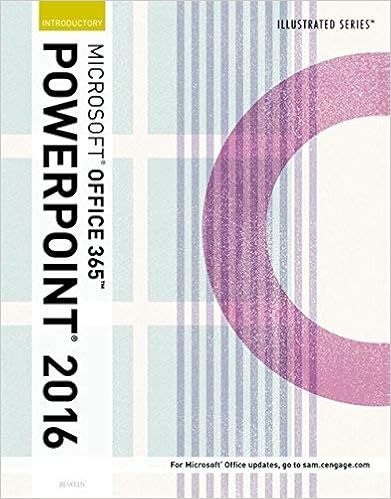# Fill in the intercept and slope values to correctly

• Homework Help
• 24
• 100% (63) 63 out of 63 people found this document helpful

This preview shows page 4 - 8 out of 24 pages.

##### We have textbook solutions for you!
The document you are viewing contains questions related to this textbook.The document you are viewing contains questions related to this textbook.
Chapter PPM2 / Exercise 21
Illustrated Microsoft Office 365 & PowerPoint 2016: Introductory
BeskeenExpert Verified
8. Fill in the intercept and slope values to correctly complete the newAE equation: AE =
YTable for Individual Question FeedbackPoints Earned: 3.0/3.0
##### We have textbook solutions for you!
The document you are viewing contains questions related to this textbook.The document you are viewing contains questions related to this textbook.
Chapter PPM2 / Exercise 21
Illustrated Microsoft Office 365 & PowerPoint 2016: Introductory
BeskeenExpert Verified
0.609. The newvalue of equilibrium output (Y) = Table for Individual Question FeedbackPoints Earned: 3.0/3.0
10. The newvalue of equilibrium consumption (C) = Feedback: NOTE:There is no graphing component to be collected for this homework. However, you shouldbe comfortable creating two separate graphs from the above questions in part 1. You should be able to:1. Graph the consumption function both before and after the shock on one graph, and also plot equilibrium consumption and output values both before and after the shock. 2.Graph the AE equation both before and after the shock on one graph, and also plot equilibrium output Y both before and after the shock.
Table for Individual Question FeedbackPoints Earned: 0.0/3.0
11. NOTE:There is no graphing component to be collected for this homework. However, you shouldbe comfortable creating two separate graphs from the above questions. You should be able to:1. Graph the consumption function both before and after the shock on one graph, and also plot equilibrium consumption and output values both before and after the shock. 2.Graph the AE equation both before and after the shock on one graph, and also plot equilibrium output Y both before and after the shock.Are your results above consistent with the new economy?
Table for Individual Question FeedbackPoints Earned: 0.0/3.0
12. Part 2 (9 questions at 3 points each for 27 points total) Pretend that you have a lemonade stand and that the demand for lemonade in your neighborhood is estimated to be: Q = 60 - 100 P Just like in the lecture, you get all the materials to make the lemonade for free so we assume
that the costs of production are zero. Your goal, your objective, is to maximize profits which is the same as maximizing total revenue given the zero cost assumption.The profit (revenue) maximizing price is cents. (don’t use a decimal point in this answer!)Table for Individual Question FeedbackPoints Earned: 3.0/3.0
•••